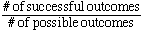I'm Kate Walace It's a 9th grade question and I am a student. The question is on Probability: If I have a spinner and then spinner has the numbers 1,2,3,4 on it and it is spun 3 times, what is the probability that the spinner will stop on 4,3,and 1 ? In that order? Can you help? If so thank you. Kate Hi Kate, Recall that the probability of an outcome of an experiment is:For the spinner with numbers 1, 2, 3 and 4 there are 4 possible outcomes. A successful outcome such as in your question would be spinning a certain number. For example there would be one successful outcome for spinning a 1, that would be landing on the 1. So for your question, the probability of spinning a 4 on the first spin is 1/4, of spinning a 3 on the second spin is 1/4 and of spinning a 1 on the third spin is 1/4. Since you need all three of these to happen, that is, you need: 4 on the 1st spin AND 3 on the 2nd spin AND 1 on the 3rd spin The answer is found by multiplying the three probabilities together to get 1/64. If we list the entire sample space for the three spins we would see that only one of the 64 possible outcomesĘcorresponds to 4, 3 and 1 in that order. Hope this helps, Leeanne Go to Math Central